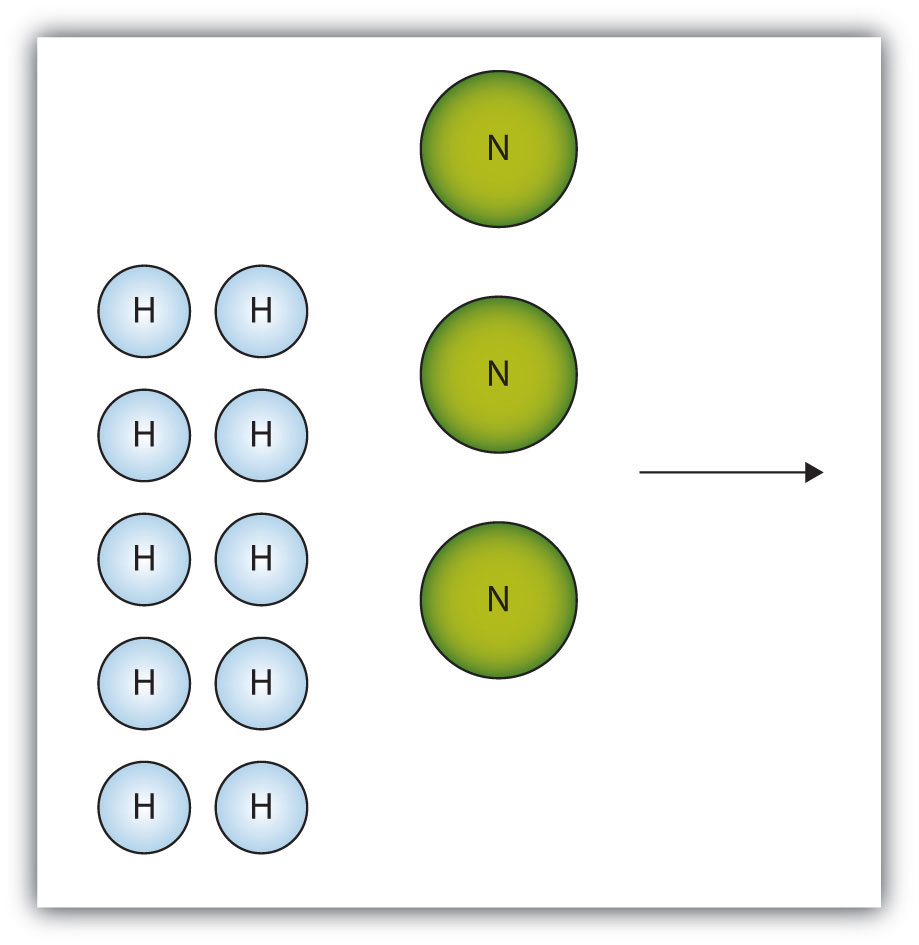# 7: Stoichiometry

$$\newcommand{\vecs}{\overset { \rightharpoonup} {\mathbf{#1}} }$$ $$\newcommand{\vecd}{\overset{-\!-\!\rightharpoonup}{\vphantom{a}\smash {#1}}}$$$$\newcommand{\id}{\mathrm{id}}$$ $$\newcommand{\Span}{\mathrm{span}}$$ $$\newcommand{\kernel}{\mathrm{null}\,}$$ $$\newcommand{\range}{\mathrm{range}\,}$$ $$\newcommand{\RealPart}{\mathrm{Re}}$$ $$\newcommand{\ImaginaryPart}{\mathrm{Im}}$$ $$\newcommand{\Argument}{\mathrm{Arg}}$$ $$\newcommand{\norm}{\| #1 \|}$$ $$\newcommand{\inner}{\langle #1, #2 \rangle}$$ $$\newcommand{\Span}{\mathrm{span}}$$ $$\newcommand{\id}{\mathrm{id}}$$ $$\newcommand{\Span}{\mathrm{span}}$$ $$\newcommand{\kernel}{\mathrm{null}\,}$$ $$\newcommand{\range}{\mathrm{range}\,}$$ $$\newcommand{\RealPart}{\mathrm{Re}}$$ $$\newcommand{\ImaginaryPart}{\mathrm{Im}}$$ $$\newcommand{\Argument}{\mathrm{Arg}}$$ $$\newcommand{\norm}{\| #1 \|}$$ $$\newcommand{\inner}{\langle #1, #2 \rangle}$$ $$\newcommand{\Span}{\mathrm{span}}$$

## Stoichiometric Reactions

Exercise $$\PageIndex{1.1}$$

For the balanced chemical equation

Al(s) + 3Ag+(aq) → Al3+(aq) + 3Ag(s)

how many moles and grams of Ag are produced when 0.661 mol of Al are reacted?

$$0.661 mol Al*\left ( \frac{3 mol Ag}{1 mol Al} \right )= 1.98 mol Ag$$

$$0.661 mol Al*\left ( \frac{3 mol Ag}{1 mol Al} \right )*\left ( \frac{107.8682 g Ag}{1 mol Ag} \right )=214 grams Ag$$

Exercise $$\PageIndex{1.2}$$

For the balanced chemical reaction

4NH3(g) + 5O2(g) → 4NO(g) + 6H2O(ℓ)

how many moles and grams of H2O are produced when 0.669 mol of NH3 react?

$.669molNH_3\left ( \frac{6molH_2O}{4molNH_3} \right )=1.00molH_2O$

1.00 mol H2O, 18.0 grams of H2O

Exercise $$\PageIndex{1.3}$$

For the balanced chemical reaction

4NaOH(aq) + 2S(s) + 3O2(g) → 2Na2SO4(aq) + 2H2O(ℓ)

how many moles and grams of Na2SO4 are formed when 1.22 grams of O2 react?

$$1.22 g O_{2}*\left ( \frac{1 mol O_{2}}{31.988 g O_{2}} \right )*\left ( \frac{2 mol Na_{2}SO_{4}}{3 mol O_{2}} \right )= 0.0254 mol Na_{2}SO_{4}$$

$$1.22 g O_{2}*\left ( \frac{1 mol O_{2}}{31.988 g O_{2}} \right )*\left ( \frac{2 mol Na_{2}SO_{4}}{3 mol O_{2}} \right )*\left ( \frac{142.04314 g Na_{2}SO_{4}}{1 mol Na_{2}SO_{4}} \right )=3.61 g Na_{2}SO_{4}$$

Exercise $$\PageIndex{1.4}$$

For the balanced chemical reaction

4KO2(s) + 2CO2(g) → 2K2CO3(s) + 3O2(g)

determine the number of moles  and grams of both products formed when 6.88 grams of KO2 react.

Moles: 0.0484 mol K2CO3, 0.0726 mol O2

Grams: 6.69 grams K2CO3, 2.32 grams O2

## Theoretical Yield, Limiting and Excess Reagents

Exercise $$\PageIndex{2.1}$$

The box below shows a group of nitrogen and hydrogen molecules that will react to produce ammonia, NH3. What is the limiting reagent?Nitrogen is the limiting reagent.

Exercise $$\PageIndex{2.2}$$

Given the statement “20.0 g of methane is burned in excess oxygen,” is it obvious which reactant is the limiting reagent?

Yes; methane is the limiting reagent.

Exercise $$\PageIndex{2.3}$$

Acetylene (C2H2) is formed by reacting 7.08 g of C and 4.92 g of H2.

2C(s) + H2(g) → C2H2(g)

What is the limiting reagent? How much of the other reactant is in excess?

C is the limiting reagent; 4.33 g of H2 are left over.

Exercise $$\PageIndex{2.4}$$

To form the precipitate PbCl2, 2.88 g of NaCl and 7.21 g of Pb(NO3)2 are mixed in solution. How much precipitate is formed? How much of which reactant is in excess?

6.06 g of PbCl2 are formed; 0.33 g of NaCl is left over.

## Percent Yield

Exercise $$\PageIndex{3.1}$$

1. What is the difference between the theoretical yield and the actual yield?
2. What is the difference between the actual yield and the percent yield?

1. Theoretical yield is what you expect stoichiometrically from a chemical reaction; actual yield is what you actually get from a chemical reaction.
2. Actual yield is what you actually get from a chemical reaction; percent yield is the percent ratio of actual yield to the theoretical yield, meaning it is a ratio that determines the accuracy of the actual yield from an experiment.

Exercise $$\PageIndex{3.2}$$

A worker isolates 2.675 g of SiF4 after reacting 2.339 g of SiO2 with HF. What are the theoretical yield and the actual yield? What is the percent yield?

SiO2(s) + 4HF(g) → SiF4(g) + 2H2O(ℓ)

theoretical yield = 4.052 g; actual yield = 2.675 g

percent yield = 66.02%

Exercise $$\PageIndex{3.3}$$

A chemist decomposes 1.006 g of NaHCO3 and obtains 0.0334 g of Na2CO3. What are the theoretical yield and the actual yield? What is the percent yield?

2NaHCO3(s) → Na2CO3(s) + H2O(ℓ) + CO2(g)

theoretical yield = 0.635 g; actual yield = 0.0334 g

percent yield = 5.26%

## Mole to Mole Conversion

Exercise $$\PageIndex{3.3}$$

Analine (C6H5NH2) can be fromend from nitro benzene (C6H5NO2) by the following equation:

4C6H5NO2 + 9Fe + 4H2O  --->   4C6H5NH2 + 3 Fe3O4

1.a) Write the conversion factor converting moles iron to moles analine.

1.b) How many moles of analine would be formed if 3.78 moles of iron was consumed?

1.c )Write the conversion factor converting moles nitro benzene to moles Fe3O4.

1.d) Write the conversion factor describing the ration fo the consumption of iron to the consumption of nitrobenzene.

1.e) How many moles of nitrobenzene would be needed to produce 4.678 Mmoles of Fe3O4?

1.f) How many moles of iron would be needed to consume 3.56x1022 molecules of nitrobenzene?

1.g)  How many atoms of iron are consumed if 3.59nmoles of water are consumed?

$$(\frac{4\;mol\;C_6H_5NH_2}{9\;mol\;Fe})$$

$$3.78mol\;Fe\;(\frac{4\;mol\;C_6H_5NH_2}{9\;mol\;Fe})\;=\;1.68\;mol\;C_6H_5NH_2$$

$$(\frac{3\;mol\;Fe_3O_4}{4\;mol\;C_6H_5NO_2})$$

$$(\frac{9\;mol\;Fe}{4\;mol\;C_6H_5NO_2})$$

$$4.678Mmole\;Fe_3O_4(\frac{10^6\;mole\;Fe_3O_4}{Mmol\;Fe_3O_4})(\frac{4\;mol\;C_6H_5NO_2}{3\;mol\;Fe_3O_4})$$

$$=\;6.237\;x\;10^6\;mol\; C_6H_5NO_2\; or \;6.237\;Mmol\;C_6H_5NO_2$$

$$3.56\;\times\;10^{22}\;molecule\;C_6H_5NO_2\;(\frac{mol\;C_6H_5NO_2}{6.022\;\times\;10^{23}\;molecule})(\frac{9\;mol\;Fe}{4\;mol\; C_6H_5NO_2 })$$

$$3.59\;nmol\;H_2O\;(\frac{10^{-9}mol\;H_2O}{nmol})(\frac{9\;mol\;Fe}{4\;mol\;H_2O})(\frac{6.022\;\times\;10^{23}\;atom\;Fe}{Fe})\;=\;4.86\; \times \;atom\;Fe$$

Exercise $$\PageIndex{3.3}$$

Analine (C6H5NH2) can be fromend from nitro benzene (C6H5NO2) by the following equation:

4C6H5NO2 + 9Fe + 4H2O  --->   4C6H5NH2 + 3 Fe3O4

2.a. How many grams of analine would be formed if 3.78moles of iron was consumed?

2.b.   How many grams of nitrobenzene would be needed to produce 4.678 kg of Fe3O4?

2.c.  How many grams of iron would be needed to consume 3.56x1022 molecules of nitrobenzene?

$$3.78\;mol\;Fe\;(\frac{4\;mol\;C_6H_5NH_2}{9\;mol\;Fe})(\frac{93.121g\;C_6H_5NH_2}{mol})\;=\;156g\;C_6H_5NH_2$$

$$4.678kg\;Fe_3O_4\;(\frac{10^3\;g\;Fe_3O_4}{kg})(\frac{mol\;Fe_3O_4}{2.31.535\;g\;Fe_3O_4})(\frac{4\;mol\;C_6H_5NH_2}{3\;mol\;Fe_3O_4})(\frac{123.105g\; C_6H_5NH_2}{mol})$$

$$=\;3.316x10^3\;g\; C_6H_5NO_2\;or\;3.316kg\;C_6H_5N)_2$$

$$(\frac{3\;mol\;Fe_3O_4}{4\;mol\;C_6H_5NO_2})$$

Exercise $$\PageIndex{3.3}$$

Analine (C6H5NH2) can be fromend from nitro benzene (C6H5NO2) by the following equation:

4C6H5NO2 + 9Fe + 4H2O  --->   4C6H5NH2 + 3 Fe3O4

2.a. How many grams of analine would be formed if 3.78moles of iron was consumed?

2.b.   How many grams of nitrobenzene would be needed to produce 4.678 kg of Fe3O4?

2.c.  How many grams of iron would be needed to consume 3.56x1022 molecules of nitrobenzene?

$$3.78\;mol\;Fe\;(\frac{4\;mol\;C_6H_5NH_2}{9\;mol\;Fe})(\frac{93.121g\;C_6H_5NH_2}{mol})\;=\;156g\;C_6H_5NH_2$$

$$4.678kg\;Fe_3O_4\;(\frac{10^3\;g\;Fe_3O_4}{kg})(\frac{mol\;Fe_3O_4}{2.31.535\;g\;Fe_3O_4})(\frac{4\;mol\;C_6H_5NH_2}{3\;mol\;Fe_3O_4})(\frac{123.105g\; C_6H_5NH_2}{mol})$$

$$=\;3.316x10^3\;g\; C_6H_5NO_2\;or\;3.316kg\;C_6H_5N)_2$$

$$(\frac{3\;mol\;Fe_3O_4}{4\;mol\;C_6H_5NO_2})$$